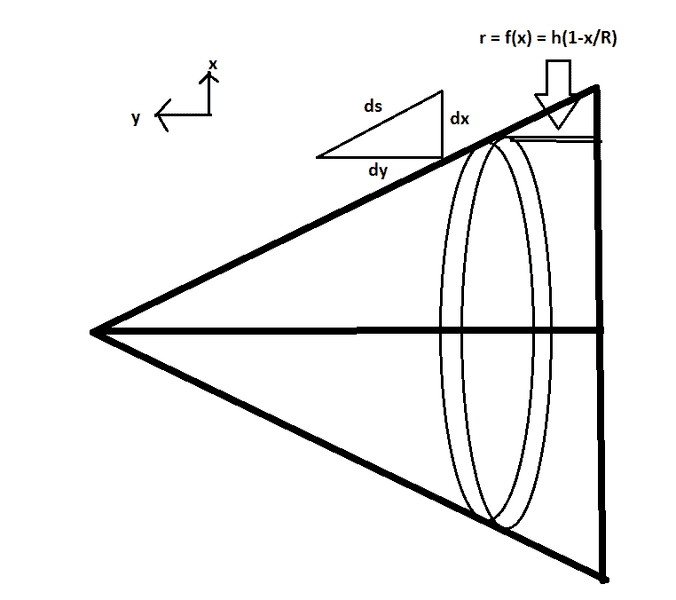# Moment of Inertia of a hollow cone about its base

## Homework Statement

Show that the moment of inertia of a hollow cone of mass M, radius R, and height h about its base is ##\frac{1}{4}M(R^2+2h^2)##

## Homework Equations

##I=\int r^2dm##
where r is the perpendicular distance from the axis

Surface Area of a cone ##= \pi R (R^2+h^2)^{1/2}##

## The Attempt at a Solution

[/B]
Mass density ##\sigma = \frac{M}{SA} = \frac{M}{\pi R(R^2+h^2)^{1/2}}##

Now ##I = \int r^2dm = \int r^2\sigma dA = \sigma \int r^2dA##Now the distance from its base is given by ##r = r(x) = h(1-x/R)## and from the image I made it appears the area element is simply ##dA = 2\pi xds##, and ##ds = \sqrt{dx^2+dy^2} = \sqrt{1+y'(x)^2}dx##. Trivially ##y'(x)^2 = (h/R)^2## giving ##dA = 2\pi x \sqrt{1+(h/R)^2}dx## and $$I = \sigma \int r^2dA =\sigma \int_0^R h^2\left(1-\frac{x}{R}\right)^22\pi x \sqrt{1+\left(\frac{h}{R}\right)^2}dx \\ I = 2\pi\sigma h^2\sqrt{1+\left(\frac{h}{R}\right)^2}\int_0^R \left(1-\frac{x}{R}\right)^2 xdx$$

which clearly isn't going to give the answer since there's no way ##\sqrt{1+(h/R)^2}## will be cancelled

## Answers and Replies

BvU
Science Advisor
Homework Helper
You sure ? What about the integral to calculate ##\sigma## ?

Ray Vickson
Science Advisor
Homework Helper
Dearly Missed

## Homework Statement

Show that the moment of inertia of a hollow cone of mass M, radius R, and height h about its base is ##\frac{1}{4}M(R^2+2h^2)##

## Homework Equations

##I=\int r^2dm##
where r is the perpendicular distance from the axis

Surface Area of a cone ##= \pi R (R^2+h^2)^{1/2}##

## The Attempt at a Solution

[/B]
Mass density ##\sigma = \frac{M}{SA} = \frac{M}{\pi R(R^2+h^2)^{1/2}}##

Formulas for surface area are poorly presented on the web, and some of them seem wrong. The more credible sources give
$$\text{SA} = \pi R \left( R + \sqrt{R^2+h^2} \right),$$
but one should make absolutely sure by calculating the relevant integral.

•Potatochip911
Formulas for surface area are poorly presented on the web, and some of them seem wrong. The more credible sources give
$$\text{SA} = \pi R \left( R + \sqrt{R^2+h^2} \right),$$
but one should make absolutely sure by calculating the relevant integral.

You sure ? What about the integral to calculate ##\sigma## ?

Whoops, I forgot to mention it's an open ended cone. I.e. There is no base portion ##pi R^2##

BvU
Science Advisor
Homework Helper
Basically you have to calculate $$\int r^2 dm\over \int dm$$

•Potatochip911
Basically you have to calculate $$\int r^2 dm\over \int dm$$

Could you elaborate on why I need to calculate this quantity when moment of inertia is given by ##I = \int r^2 dm##?

Ray Vickson
Science Advisor
Homework Helper
Dearly Missed

## Homework Statement

Show that the moment of inertia of a hollow cone of mass M, radius R, and height h about its base is ##\frac{1}{4}M(R^2+2h^2)##

## Homework Equations

##I=\int r^2dm##
where r is the perpendicular distance from the axis

Surface Area of a cone ##= \pi R (R^2+h^2)^{1/2}##

## The Attempt at a Solution

[/B]
Mass density ##\sigma = \frac{M}{SA} = \frac{M}{\pi R(R^2+h^2)^{1/2}}##

Now ##I = \int r^2dm = \int r^2\sigma dA = \sigma \int r^2dA##
View attachment 109197

Now the distance from its base is given by ##r = r(x) = h(1-x/R)## and from the image I made it appears the area element is simply ##dA = 2\pi xds##, and ##ds = \sqrt{dx^2+dy^2} = \sqrt{1+y'(x)^2}dx##. Trivially ##y'(x)^2 = (h/R)^2## giving ##dA = 2\pi x \sqrt{1+(h/R)^2}dx## and $$I = \sigma \int r^2dA =\sigma \int_0^R h^2\left(1-\frac{x}{R}\right)^22\pi x \sqrt{1+\left(\frac{h}{R}\right)^2}dx \\ I = 2\pi\sigma h^2\sqrt{1+\left(\frac{h}{R}\right)^2}\int_0^R \left(1-\frac{x}{R}\right)^2 xdx$$

which clearly isn't going to give the answer since there's no way ##\sqrt{1+(h/R)^2}## will be cancelled

Yes, it will cancel because it appears as well in the denominator of your equation for ##\sigma##.

•Potatochip911
Yes, it will cancel because it appears as well in the denominator of your equation for ##\sigma##.

Hmm, evaluating that integral gives ##I= Mh^2/6## which unfortunately isn't the correct answer